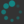## Exercises

1. Suppose that $X$ deformation retracts to a point $x\in X.$ Show that for each neighborhood $U$ of $x$ in $X,$ there exists a neighborhood $V\subset U$ of $x$ such that $V\hookrightarrow U$ is nulhomotopic.
2. Show that a retract of a contractible space is contractible.